# Chapter 4: Training Neural Networks¶

By Tomas Beuzen 🚀## Chapter Learning Objectives¶

• Explain how backpropagation works at a high level.

• Describe the difference between training loss and validation loss when creating a neural network.

• Identify and describe common techniques to avoid overfitting/apply regularization to neural networks, e.g., early stopping, drop out, L2 regularization.

• Use PyTorch to develop a fully-connected neural network and training pipeline.

## Imports¶

import numpy as np
import pandas as pd
import torch
from torch import nn
from torchvision import transforms, datasets, utils
from torch.utils.data import DataLoader, TensorDataset
from utils.plotting import *


## 1. Differentiation, Backpropagation, Autograd¶

In previous chapters we’ve discussed optimization algorithms like gradient descent, stochastic gradient descent, ADAM, etc. These algorithms need the gradient of the loss function w.r.t the model parameters to optimize the parameters:

$\begin{split}\nabla \mathscr{L}(\mathbf{w}) = \begin{bmatrix} \frac{\partial \mathscr{L}}{\partial w_1} \\ \frac{\partial \mathscr{L}}{\partial w_2} \\ \vdots \\ \frac{\partial \mathscr{L}}{\partial w_d} \end{bmatrix}\end{split}$

We’ve been able to calculate the gradient by hand for things like linear regression and logistic regression. But how would you work out the gradient for this very simple network for regression: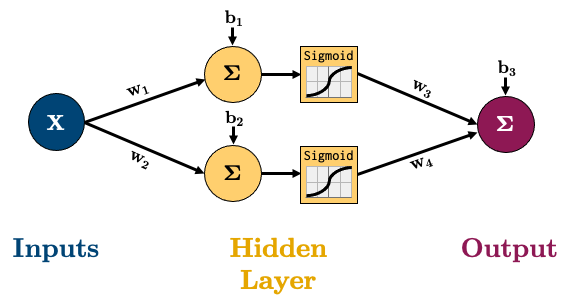The equation for calculating the output of that network is below, it’s the linear layers and activation functions (Sigmoid in this case) recursively stuck together:

$S(x)=\frac{1}{1+e^{-x}}$
$\hat{y}=w_3S(w_1x+b_1) + w_4S(w_2x+b_2) + b_3$

So how would we calculate the gradient of say the MSE loss w.r.t to all our parameters?

$\mathscr{L}(\mathbf{w}) = \frac{1}{n}\sum^{n}_{i=1}(y_i-\hat{y_i})^2$
$\begin{split}\nabla \mathscr{L}(\mathbf{w}) = \begin{bmatrix} \frac{\partial \mathscr{L}}{\partial w_1} \\ \frac{\partial \mathscr{L}}{\partial w_2} \\ \vdots \\ \frac{\partial \mathscr{L}}{\partial w_d} \end{bmatrix}\end{split}$

We have 3 options:

1. Symbolic differentiation: i.e., “do it by hand” like we learned in calculus.

2. Numerical differentiation: for example, approximating the derivative using finite differences $$\frac{df(x)}{dx} \approx \frac{f(x+h)-f(x)}{h}$$.

3. Automatic differentiation: the “best of both worlds”.

We’ll be looking at option 3 Automatic Differentiation (AD) here, as we use a particular flavour of AD called “backpropagation” to train neural networks. But if you’re interested in learning more about the other methods, see Appendix C: Computing Derivatives.

### 1.1. Backpropagation¶

Backpropagation is the algorithm we use to compute the gradients needed to train the parameters of a neural network. In backpropagation, the main idea is to decompose our network into smaller operations with simple, codeable derivatives. We then combine all these smaller operations together with the chain rule. The term “backpropagation” stems from the fact that we start at the end of our network and then propagate backwards. I’m going to go through a short example based on this network: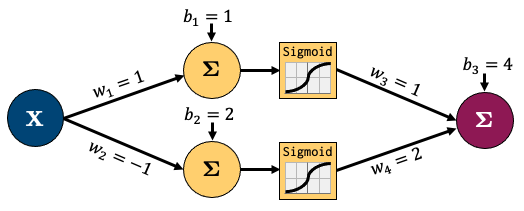Let’s decompose that into smaller operations. I’ve introduced some new variables to hold intermediate states $$z_i$$ (node output before activation) and $$a_i$$ (node output after activation). I’ll also feed in one sample data point (x, y) = (1, 3) and am showing intermediate outputs in green and the final loss in red. This is called the “forward pass” step - where I feed in data and calculate outputs from left to right: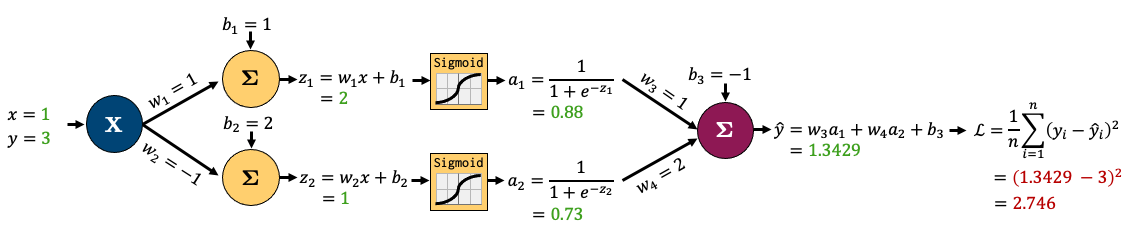Now let’s zoom in to the outpout node and calculate the gradients for just the parameters connected to that node. It looks complicated but the derivatives are very simple - take some time to examine this figure and you’ll see!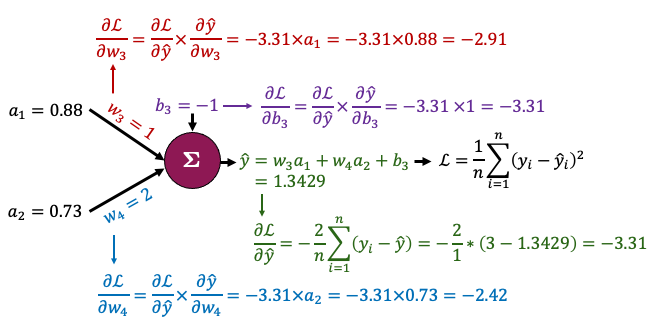That all boils down to this: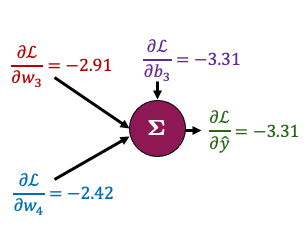Now, the beauty of backpropagation is that we can use these results to easily calculate derivatives earlier in the network using the chain rule. I’ll do that for $$b_1$$ and $$b_2$$ below. Once again, it looks complicated, but we’re simply combining a bunch of small, simple derivatives with the chain rule: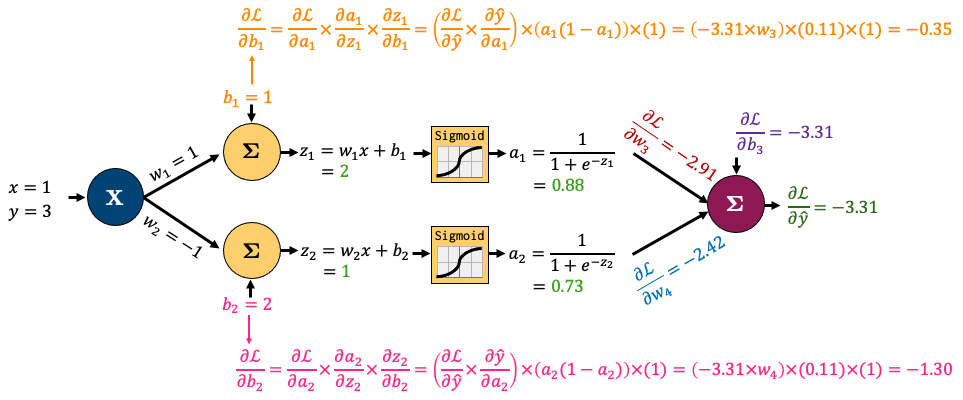I’ve left calculating the gradients of $$w_1$$ and $$w_2$$ up to you. All the gradients for the network boil down to this: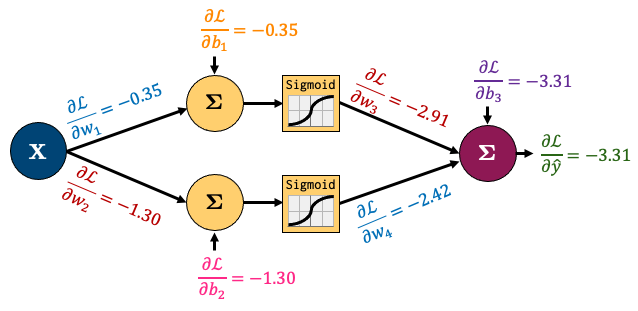So summarising the process:

1. We “forward pass” some data through our network

2. We “backpropagate” the error through the network to calculate gradients

Luckily, you’ll never do this by hand again, because torch.autograd does all this for us!

torch.autograd is PyTorch’s automatic differentiation engine which helps us implement backpropagation. In plain English: torch.autograd automatically calculates and stores derivatives for your network. Consider our simple network above:class network(torch.nn.Module):
def __init__(self, input_size, hidden_size, output_size):
super().__init__()
self.hidden = torch.nn.Linear(input_size, hidden_size)
self.output = torch.nn.Linear(hidden_size, output_size)

def forward(self, x):
x = self.hidden(x)
x = torch.sigmoid(x)
x = self.output(x)
return x

model = network(1, 2, 1)  # make an instance of our network
model.state_dict()['hidden.weight'][:] = torch.tensor([, [-1]])  # fix the weights manually based on the earlier figure
model.state_dict()['hidden.bias'][:] = torch.tensor([1, 2])
model.state_dict()['output.weight'][:] = torch.tensor([[1, 2]])
model.state_dict()['output.bias'][:] = torch.tensor([-1])
x, y = torch.tensor([1.0]), torch.tensor([3.0])  # our x, y data


Now let’s check the gradient of the bias of the output node:

print(model.output.bias.grad)

None


It’s currently None!

PyTorch is tracking the operations in our network and how to calculate the gradient (more on that a bit later), but it hasn’t calculated anything yet because we don’t have a loss function and we haven’t done a forward pass to calculate the loss so there’s nothing to backpropagate yet!

Let’s define a loss now:

criterion = torch.nn.MSELoss()


Now we can force Pytorch to “backpropagate” the errors, like we just did by hand earlier by:

1. Doing a “forward pass” of our (x, y) data and calculating the loss;

2. “Backpropagating” the loss by calling loss.backward()

loss = criterion(model(x), y)
loss.backward()  # backpropagates the error to calculate gradients!


Now let’s check the gradient of the bias of the output node ($$\frac{\partial \mathscr{L}}{\partial b_3}$$):

print(model.output.bias.grad)

tensor([-3.3142])


It matches what we calculated earlier!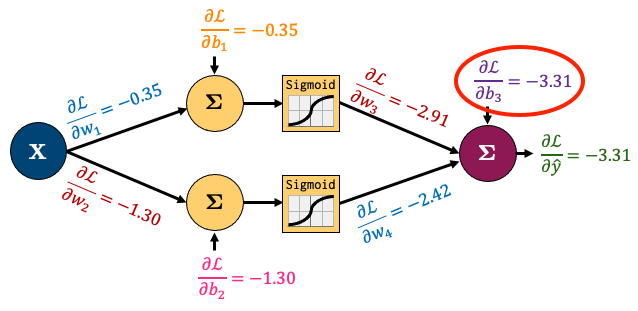That is just so fantastic! In fact, we can make sure that all our gradients match what we calculated by hand:

print("Hidden Layer Gradients")
print()

Hidden Layer Gradients
Bias: tensor([-0.3480, -1.3032])
Weights: tensor([-0.3480, -1.3032])

Bias: tensor([-3.3142])
Weights: tensor([-2.9191, -2.4229])


Now that we have the gradients, what’s the next step? We use our optimization algorithm to update our weights! These are our current weights:

model.state_dict()

OrderedDict([('hidden.weight',
tensor([[ 1.],
[-1.]])),
('hidden.bias', tensor([1., 2.])),
('output.weight', tensor([[1., 2.]])),
('output.bias', tensor([-1.]))])


To optimize them, we:

1. Define an optimizer;

2. Ask it to update our weights based on our gradients using optimizer.step().

optimizer = torch.optim.SGD(model.parameters(), lr=0.1)
optimizer.step()


Our weights should now be different:

model.state_dict()

OrderedDict([('hidden.weight',
tensor([[ 1.0348],
[-0.8697]])),
('hidden.bias', tensor([1.0348, 2.1303])),
('output.weight', tensor([[1.2919, 2.2423]])),
('output.bias', tensor([-0.6686]))])


Amazing!

One last thing for you to know: Pytorch does not automatically clear the gradients after using them. So if I call loss.backward() again, my gradients accumulate:

optimizer.zero_grad()  # <- I'll explain this in the next cell
for _ in range(1, 6):
loss = criterion(model(x), y)
loss.backward()
print(f"b3 gradient after call {_} of loss.backward():", model.hidden.bias.grad)

b3 gradient after call 1 of loss.backward(): tensor([-0.1991, -0.5976])
b3 gradient after call 2 of loss.backward(): tensor([-0.3983, -1.1953])
b3 gradient after call 3 of loss.backward(): tensor([-0.5974, -1.7929])
b3 gradient after call 4 of loss.backward(): tensor([-0.7966, -2.3906])
b3 gradient after call 5 of loss.backward(): tensor([-0.9957, -2.9882])


Our gradients are accumulating each time we call loss.backward()! So we need to tell Pytorch to “zero the gradients” each iteration using optimizer.zero_grad():

for _ in range(1, 6):
optimizer.zero_grad()  # <- don't forget this!!!
loss = criterion(model(x), y)
loss.backward()
print(f"b3 gradient after call {_} of loss.backward():", model.hidden.bias.grad)

b3 gradient after call 1 of loss.backward(): tensor([-0.1991, -0.5976])
b3 gradient after call 2 of loss.backward(): tensor([-0.1991, -0.5976])
b3 gradient after call 3 of loss.backward(): tensor([-0.1991, -0.5976])
b3 gradient after call 4 of loss.backward(): tensor([-0.1991, -0.5976])
b3 gradient after call 5 of loss.backward(): tensor([-0.1991, -0.5976])


Note: you might wonder why PyTorch behaves like this. Well, there are some cases we might want to accumulate the gradient. For example, if we want to calculate the gradients over several batches before updating our weights. But don’t worry about that for now - most of the time, you’ll want to be “zeroing out” the gradients each iteration.

### 1.3. Computational Graph (Optional)¶

PyTorch’s autograd basically keeps a record of our data and network operations in a computational graph. That’s beyond the scope of this chapter, but if you’re interested in learning more, I recommend this excellent video. Also, torchviz is a useful package to look at the “computational graph” PyTorch is building for us under the hood:

from torchviz import make_dot
make_dot(model(torch.rand(1, 1)))## 2. Training Neural Networks¶

The big takeaway from the last section is that PyTorch’s autograd takes care of the gradients for us. We just need to put all the pieces together properly. Remember the below trainer() function I used last chapter to train my network. Now we know what all this means!

def trainer(model, criterion, optimizer, dataloader, epochs=5):
"""Simple training wrapper for PyTorch network."""

train_loss = []
for epoch in range(epochs):  # for each epoch
losses = 0
for X, y in dataloader:  # for each batch
optimizer.zero_grad()       # Zero all the gradients w.r.t. parameters
y_hat = model(X).flatten()  # Forward pass to get output
loss = criterion(y_hat, y)  # Calculate loss based on output
loss.backward()             # Calculate gradients w.r.t. parameters
optimizer.step()            # Update parameters
losses += loss.item()       # Add loss for this batch to running total
train_loss.append(losses / len(dataloader))  # loss = total loss in epoch / number of batches = loss per batch
return train_loss


Notice how I calculate the loss for each epoch by summing up the loss for each batch in that epoch? I then divide the loss per epoch by total number of batches to get the average loss per batch in an epoch (I store that loss in running_losses).

Dividing by the number of batches “decouples” our loss from the batch size. So if I run another experiment with a different batch size, I’ll still be able to compare losses for that experiment with this one. We’ll explore this concept more later.

If our model is being trained correctly, our loss should go down over time. Let’s try it out with some sample data:

# Create dataset
torch.manual_seed(0)
X = torch.arange(-3, 3, 0.15)
y = X ** 2 + X * torch.normal(0, 1, (40,))
dataloader = DataLoader(TensorDataset(X[:, None], y), batch_size=1, shuffle=True)
plot_regression(X, y, y_range=[-1, 10], dy=1)